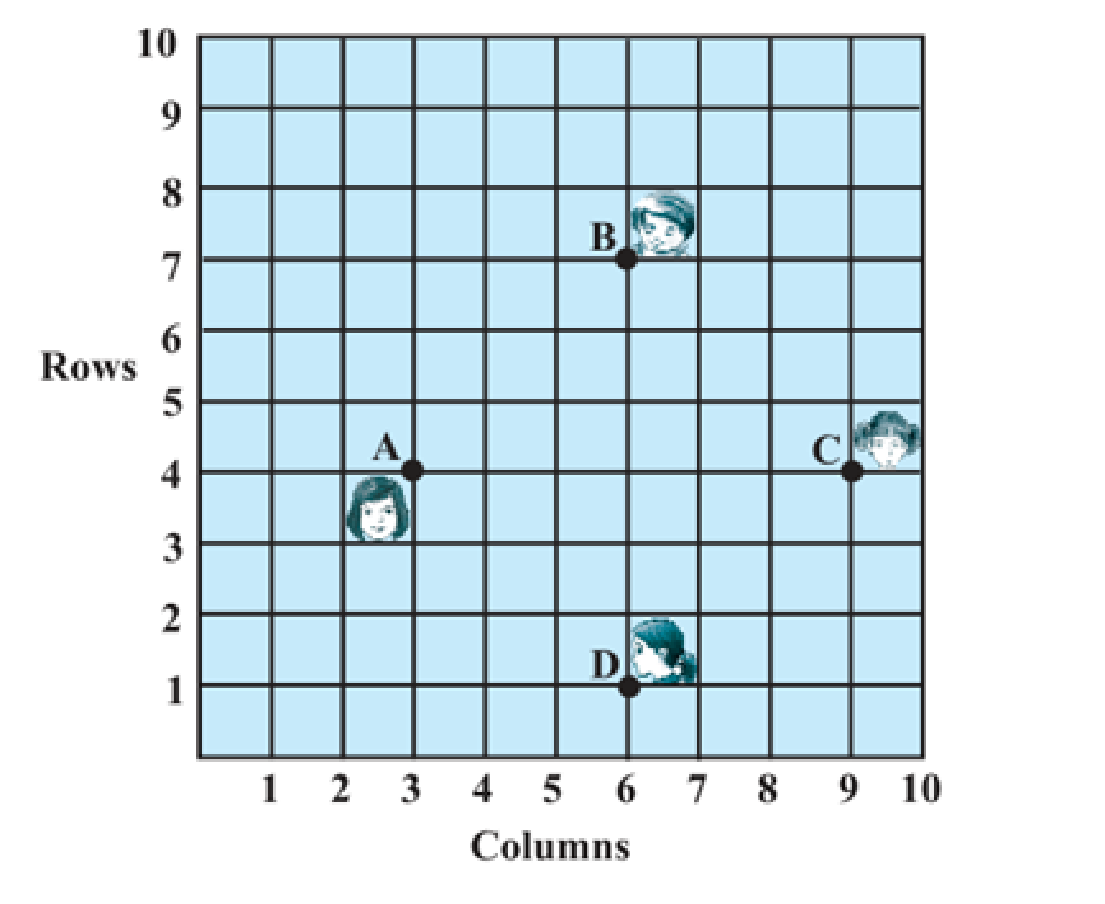# NCERT Solutions For Class 10 Math Chapter – 7 Exercise – 7.1

NCERT Solutions For Class 10 Math Chapter – 7 Coordinate Geometry Exercise – 7.1

The exercise solutions provided here are created with a vision to assist the students in their first term exam preparation. Exercise solution cover all the questions given in Exercise 7.1 of the NCERT textbook. These solutions are researched and prepared by our subject experts. Studying this study material will aid you in solving different questions on distance from a point.

Exercise solutions are provided in PDF format for your easy access and download. By studying this solution you can clear your doubts on distance calculation. By studying this study material you can get well acquainted with all important formulas. Here, you can also get knowledge of alternative methods to solve distance from a point problems.

Q1.

FIND THE DISTANCE BETWEEN THE FOLLOWING  PAIRS OF POINTS

(i) (2,3) (4,1)

• (-5,7) , (-1,3)
• (a,b) , (-a , -b)

Solution:-

Distance formula to find the distance between two points (x1 , y1) and (x2 , y2)

D = √(x2 – x1)2 + (y2 – y1)2

• D = √(4-2)2+ (1-3)2

= √8

= 2√2.

• D = √(-1 + 5)2+ (3- 7)2

= √32

= 4√2.

• D = √(-2a)2+ (-2b)2

= 2√a2 + b2

Q2. Find the distance between the points (0,0)  and (36,15) Can you now find the distance between the two towns A and B discussed in Section 7.2.

Solution:-

D = √(36 – 0)2 + (15 – 0)2

= √1521

= 39

The distance between town A and B will be 39 km.

Q3. Determine if the points ( 1, 5) (2,3) and (-2,11) are collinear.

Solution:-

AB = √(2-1)2 + (3-5)2

= √1+4

= √5.

BC = √(-2-2)2 + (-11-3)2

= √16 + 196

= √212.

CA = √(-2-1)2 + (-11-5)2

= √9 + 256

= √265

Since AB + BC ≠ CA

Therefore , the points ( 1, 5) (2,3) and (-2 , -11) are not collinear.

Q4. Check whether ( 5  , -2) (6,4) and (-7,2) are the vertices of an isosceles triangle.

Solution:-

AB = √(6-5)2 + (4+2)2

= √37

BC = √(7-6)2 + (-2-4)2

= √37

CA = √(7-5)2 + (-2+2)2

= 2

This implies whether given points are vertices of an isosceles triangle.

Q5.  In a classroom 4 friends are seated at the points A , B , C and D Champa and Chameli walk into the class and after observing for a few minutes Champa asks chameli Don’t you think ABCD is a square ? “ Chameli disagrees Using distance formula , find which of them is correct.Solution:-

AB = √(6-3)2 + (7-4)2

= √9 + 9

= 3√2

BC = √(9-6)2 + (4-7)2

= √9 + 9

= 3√2

CD = √(6-9)2 + (1-4)2

= √9 + 9

= 3√2

DA = √(6-3)2 + (1-4)2

= √9 + 9

= 3√2

AB = BC = CD = DA = 3√2

All sides are of equal length Therefore , ABCD is a square and hence , Champa was correct.

Q6. Name the type of quadrilateral  formed if any , by the following points , and give reasons for your answer:

• (-1,-2) , (1,0) , (-1,2) , (-3,0)
• (-3,5) , (3,1) , (0,3) , (-1,-4)
• (4,5) , (7,6) , (4,3) , (1,2)

Solution:-

• AB = √(1+1)2+ (0+2)2

= √4 + 4

= 2√2

BC = √(-1 – 1)2 + (2-0)2

= √4 + 4

= 2√2

CD = √(-3 + 1)2 + (0-2)2

= √4 + 4

= 2√2

DA = √(-3+1)2 + (0-2)2

= √4 + 4

= 2√2

AC = √(-1+1)2 + (2 +2)2

= √0 + 14

= 4

BD = √(-3-1)2 + (0-0)2

= √16 + 0

= 4

Square.

• AB = √(-3-3)2 + (1-5)2

= √36 + 16

= 2√13

BC = √(0-3)2 + (3-1)2

= √9+4

= √13

CD = √(-1+3)2 + (-4-5)2

= √4 + 81

= √85

Therefore , the given points cannot form a general quadrilateral.

• AB = √(7-4)2+ (6-5)2

= √9 + 1

= √10

BC = √(4-7)2 + (3-6)2

= √9 + 9

= √18

CD = √(1-4)2 + (2-3)2

= √9 + 1

= √10

= √9 + 9

= √18

AC = √(4-4)2 + (3-5)2

= √0 +  4

= 2

BD = √(1-7)2 + (2-6)2

= √36 + 16

= 2√13

Q7. Find the point on the x- axis which is equidistant from (2,-5) and (-2,9).

Solution:-

A = (x,0)   B = (2,-5)  C = (-2,9)

AB = √(2-X)2 + (-5-0)2

= √(2-x)2 + 25

AC = √(-2-X)2 + (9-0)2

= √-2 – x)2 + 81

√(2-x)2 + 25 = √(-2-x)2 + 81

• x)2+ 25 = (-2 -x)2+ 81

8x = 25 – 81

X = -7

Therefore the point is (-7,0).

Q8. Find the values of y for which the distance between the points P (2 , -3) and Q(10 , y) is 10 units

Solution:-

PQ = √(10 – 2)2 + (Y + 3)2 = 10

= √(8)2 + (Y+3)2

64 + (Y+3)2 = 100

(Y+3)2= 36

Y + 3 = 6

Y+ 3 = 6 Y + 3 = -6

Y = 3 , or  -9.

Q9. If Q (0,1) is equidistant from P(5,-3) and R(x,6) find the values of x Also find the distance QR and PR

Solution:-

PQ = √(5-0)2 + (-3-1)2

= √(-5)2 + (-4)2

= √41

QR = √(0-X)2 + (1-6)2

= √(-X)2 + (-5)2

= √X2  + 25

Use PQ = QR

√41 = √x2 + 25

Squaring both sides

41 = x2 + 25

X2 = 16

X = 4

X = 4 or x = -4

Coordinate of Point R will be R (4,6) or (-4,6)

If R (4,6) then QR

QR = √(0+4)2 + (1-6)2

= √16 + 25

= √41

PR = √(5+4)2 + (-3-6)2

= 9√2.

Q10. Find a relation between x and y such that the point (x,y) is equidistant from the point (3,6) and (-3,4).

Solution:-

√(x-3)2 + (y-6)2 = √x – (-3)2 + (y-4)2

√(x-3)2 + (y-6)2 = √(x+3)2 + (y-4)2

Squaring both sides

(x-3)2 + (y-6)2 = (x+3)2 + (y-4)2

20 = 12x + 4y

3x + y – 5y = 0